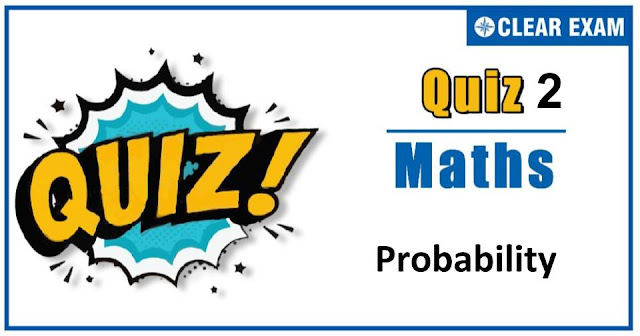## [LATEST]\$type=sticky\$show=home\$rm=0\$va=0\$count=4\$va=0

As per analysis for previous years, it has been observed that students preparing for JEE MAINS find Mathematics out of all the sections to be complex to handle and the majority of them are not able to comprehend the reason behind it. This problem arises especially because these aspirants appearing for the examination are more inclined to have a keen interest in Mathematics due to their ENGINEERING background.

Furthermore, sections such as Mathematics are dominantly based on theories, laws, numerical in comparison to a section of Engineering which is more of fact-based, Physics, and includes substantial explanations. By using the table given below, you easily and directly access to the topics and respective links of MCQs. Moreover, to make learning smooth and efficient, all the questions come with their supportive solutions to make utilization of time even more productive. Students will be covered for all their studies as the topics are available from basics to even the most advanced.

Q1. If A and B are two events such that P(A∪B)=5/6, P(A∩B)=1/3 and P(B')=1/3,then the value of P(A)is
•  1/3
•  1/4
•  1/2
•  2/3
Solution
(c) Given, 1-P(B)=1/3⇒P(B)=2/3, P(A∪B)=5/6,P(A∩B)=1/3 Now, P(A∪B)=P(A)+P(B)-P(A∩B) ⇒5/6=P(A)+2/3-1/3 ⇒P(A)=5/6-2/3+1/3=1/2

Q2.A signal which can be green or red with probability4/5 and 1/5 respectively, is received by station A and then transmitted to stationB. The probability of each station receiving the signal correctly is 3/4.If the signal received at station B is green, then the probability that the original signal green is
•  3/5
•  6/7
•  20/23
•  9/20
Q3.  The distribution of a random variable X is given below X -2 -1 0 1 2 3 P(X) 1/10 k 1/5 2k 3/10 k The value of k is
•   1/10
•  2/10
•  3/10
•  7/10
Solution
(a) We know, total probability distribution is 1. ∴ 1/10+k+1/5+2k+3/10+k=1 ⇒ 6/10+4k=1 ⇒ k=1/10

Q4. Seven white balls and three black balls are randomly placed in a row. The probability that no two black balls are placed adjacently, equals
•  1/2
•  7/15
•  2/15
•  1/13
Solution
(b) Explaination not available

Q5.There are n letters and n addressed envelopes, the probability that all the letters are not kept in the right envelope, is
•  1/n!
•  1-1/n!
•  1-1/n
•  n!
Solution
(b) Required probability=1-P(all letters in right envelope) =1-1/n!

Q6. The probability of getting a total of at least 6 in the simultaneously throw of three dice is
•  6/108
•  5/27
• 1/24
•  103/108
Solution
(d) Total number of cases=6^3=216 Let A denote the event of getting at least 6, A' will denote the event of getting less than 6 for three dice. ∴A ̅={(1,1,1),(1,12),(1,1,3,),(1,2,1),(1,3,1),(2,1,2),(1,2,2),(2,2,1),(2,1,1),(3,1,1)} ⇒n(A ̅)=10 Probability of getting less than 6=10/216=5/108 Now, P(A)=1-P(A ̅ )=1-5/108=103/108

Q7.Five boys and three girls are seated at random in a row. The probability that no boy sits between two girls is
•  1/56
•  1/8
•  3/28
•  None of these
Solution
(c) Five boys and 3 girls can sit in a row in 8 ! ways. Considering three girls as one individual. There are 6 persons, who can sit in a row in 6 ! ways. But, 3 girls can sit together in 3! Ways ∴ Number of ways in which 5 boys and 3 girls can sit in a row when 3 girls sit together =6!×3! Hence, required probability =(6!×3)/8!=3/28

Q8.If A and B are two events, then P (neither A nor B) equals
•  1-P(A∪B)
•  P(A')+P(B')
•  1-P(A)-P(B)
•  None of these
Q9.A letter is taken out at random from ‘ASSISTANT’ and another is taken out from ‘STATISTICS’. The probability that they are same letters, is
•  1/45
•  13/90
•  19/90
•  None of these
Solution
(c) Given, ASSISTANT→AA I N SSS TT→ STATISTICS→AC II SSS TTT Hence, N and C are not common Same letters can be A, I, S, T Probability of choosing ‘A’ photo Hence, required probability =1/45+1/45+1/10+1/15=19/90

Q10. The probability that a leap year selected at random will contain either 53 Thursdays or 53 Fridays, is
•  3/7
•  2/7
•  5/7
• 1/6
Solution
(a) A leap year consists of 366 days ie, 52 full weeks and two extra days. These two extra days can be any one of the following possible outcomes; (i) Monday and Tuesday, (ii) Tuesday and Wednesday, (iii) Wednesday and Thursday, (iv) Thursday and Friday, (v) Friday and Saturday, (vi) Saturday and Sunday, (vii) Sunday and Monday. Let A and B be the events that a leap year contains 53 Thursdays and 53 Fridays respectively. Then, P(A)=2/7,P(B)=2/7 and P(A∩B)=1/7 ∴ Required probability is given by P(A∪B)=P(A)+P(B)-P(A∩B) =2/7+2/7-1/7=3/7## Want to know more

Want to Know More
Please fill in the details below:

## Latest NEET Articles\$type=three\$c=3\$author=hide\$comment=hide\$rm=hide\$date=hide\$snippet=hide

Name

ltr
item
Best IIT JEE Coaching Institute in Delhi | NEET Coaching Institute in Delhi: Probability-quiz-2
Probability-quiz-2